Next: Voltage and Current Offsets Up: Analysis Using Finite Open-Loop Previous: Output Impedance

## Input Impedance

When calculating the output impedance we still assumed an infinite input impedance. In this section we will calculate the finite input impedance assuming a zero output impedance. We consider a model that assumes an internal resistor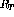connecting the inverting and non-inverting input terminals of the op-amp as shown in figure 6.31

Consider an inverting amplifier and remove the input resistor so that the input impedance can be calculated directly at the amplifier's input terminals.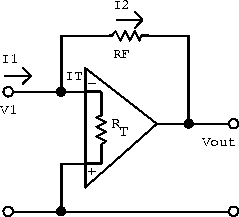Figure 6.31:  Model for calculating the input impedance of the inverting amplifier.

The input impedance is defined by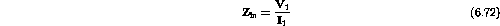and the current at the summing junction is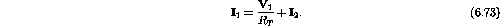The current through the feedback resistor is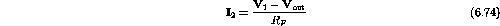and the output voltage is related to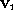by the open-loop gain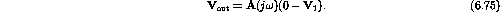The resulting input impedance is thus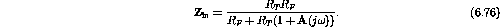For large A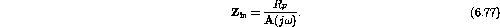The closed-loop input impedance is thus small and almost independent of the largeof the operational amplifier.

Now consider the non-inverting amplifier shown in figure 6.32.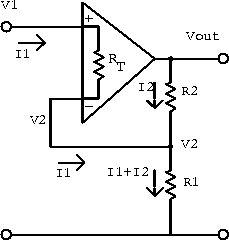Figure 6.32:  Model for calculating the input impedance of the non-inverting amplifier.

The student should calculate the input impedance by recognizing that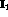is much less than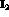, sinceis much greater than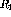or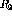. Your result should be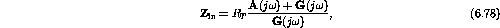where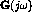is the closed-loop gain of the amplifier. Notice that in contrast to the low input impedance for the inverting amplifier, the non-inverting amplifier exhibits a closed-loop input impedance that is much larger than the open-loop value.

Doug Gingrich
Tue Jul 13 16:55:15 EDT 1999